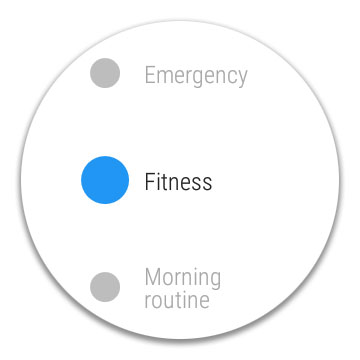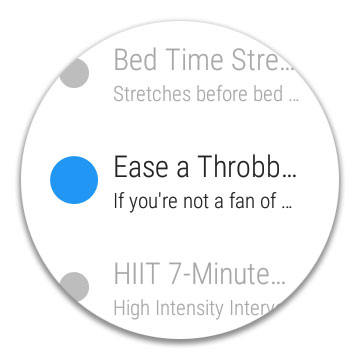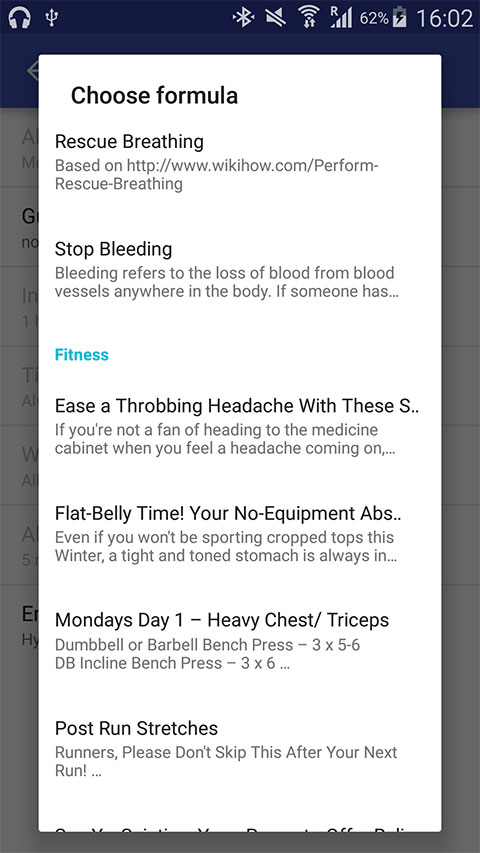GuruWear
Create new formula
Help > Manuals > GuruWear for Android API

## Choose formula

Choose formula from all installed formulas. Can be started on mobile or directly on wearable.

Intent actoion: net.guruwear.PICK_FORMULA
Available for: mobile, wearable
Extras: none
Result extra:

• net.guruwear.FORMULA_ID (string) Formula identifier
• net.guruwear.FORMULA_NAME (string) Formula name
• net.guruwear.FORMULA_DESCRIPTION (string) Formula description
• net.guruwear.FORMULA_CATEGORY (string) Formula category

### Sample code:

``````private static final int PICK_FORMULA_REQUEST = 325;

String mFormulaId;
String mFormulaName;

/**
*
* open formula choose activity
*
*/
public void pickFormula()
{
Intent i = new Intent();
i.setAction("net.guruwear.PICK_FORMULA");
startActivityForResult(i, PICK_FORMULA_REQUEST);
}

/**
*
* Get results
*
*/
@Override
protected void onActivityResult(int requestCode, int resultCode, Intent data) {

if (requestCode == PICK_FORMULA_REQUEST) {

if (resultCode == RESULT_OK) {

mFormulaId = data.getStringExtra("net.guruwear.FORMULA_ID");
mFormulaName = data.getStringExtra("net.guruwear.FORMULA_NAME");

//saveData();

} else {

mFormulaId = "";
mFormulaName = "";

//saveData();

}
}
}``````## Start formula

Start specified formula on Android Wear watch. Can be started from mobile or directly on wearable device.

Intent action: net.guruwear.RUN_FORMULA
Available for: mobile, wearable
Extras:

• net.guruwear.FORMULA_ID (string, required)
• net.guruwear.PROGRESS_OPTION (integer, optional) - determine how to deal with saved formula progress
Values:
• 0 (Progress ask) – ask to continue or restart formula with saved progress
``````Intent i = new Intent();